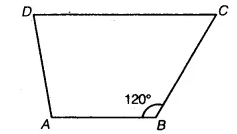# Find m∠C in the figure, if AB || DC

Find m∠C in the figure, if$\overline { AB }$ ||$\overline { DC }$.Since AB || DC and transversal BC intersects
them.
∠B + ∠C= 180°
[∵ Sum of interior angles is 180°]
⇒ 120°+ ∠C =180° A
⇒ ∠C = 180°- 120° = 60°
Hence, m∠C =60°